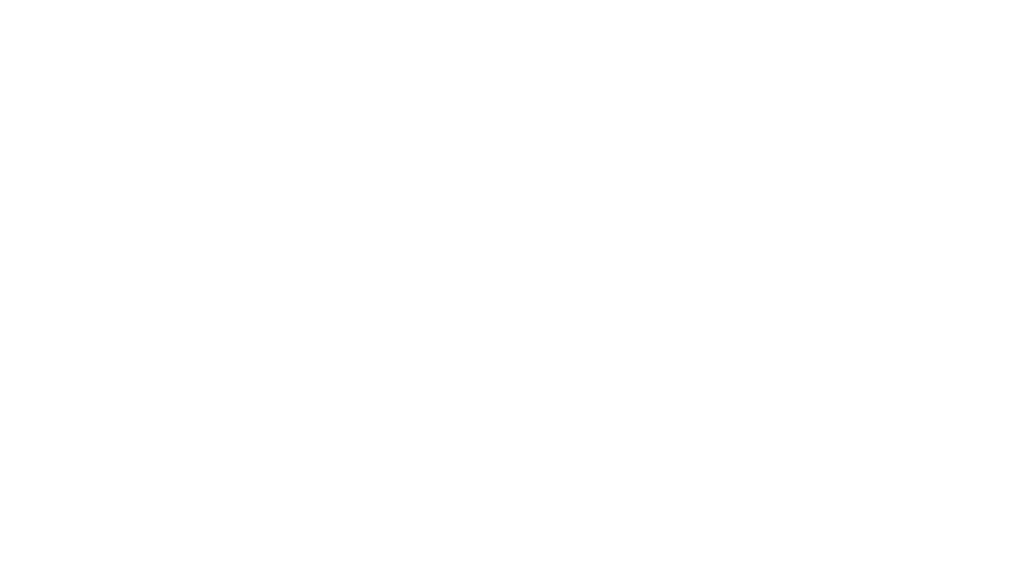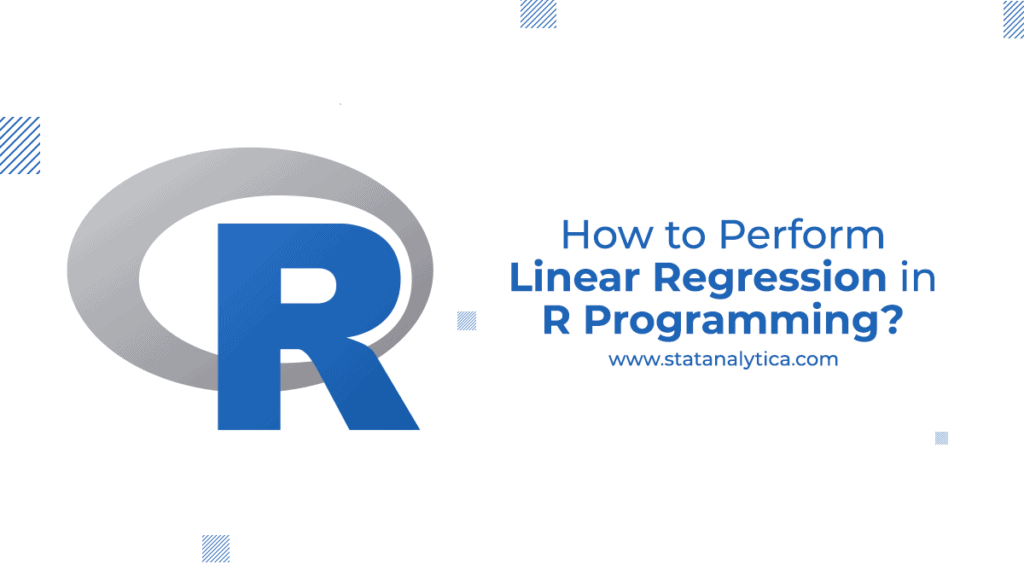# How to Perform Linear Regression in R Programming?

Linear regression is the most preferred method in statistics. It is mostly used to quantify the correlation among the single or multiple predictive and responsive variables. Simple linear regression in R is the primary form of linear regression that is also used to quantify the relationship among the single or multiple predictive and responsive variables. If you have multiple predictive variables, then it is always better to use multiple linear regression. Below we have listed how to use regression in R but before proceeding to the details, let’s take a real-life example of linear regression.

## A real-life example of linear regression

Various businesses mostly use linear regression techniques to know the relationship between revenue and advertising spending. For example, Businesses analyze the linear regression models by considering advertising spending as a predictive variable to the revenue as a response variable. Therefore, the linear regression models considered as :

where,

The β0 coefficient = Total expected revenue (In the case when advertising spends is zero.)

The β1 coefficient = Average change in the revenue (if the advertising spends increases by a single unit.)

Now, there are 3 different case related to the β1 coefficient:

1. When β1 is negative, which means that maximum advertising spends get associated with minimum revenue.
2. When β1 is nearer to zero, which means that less advertising spends cause less impact on revenue.
3. And when β1 is positive, which means that more advertising spends get associated with maximum revenue.

Therefore, it can be summarized that based on the β1 value of the models, a company can easily decide whether to increase or decrease the advertising spends.

## Simple linear regression in R

Simple linear regression allows the user to conclude and study the correction between the two quantitative (continuous) variables. Here, a variable is denoted by x and considered to be independent variables, whereas another is denoted by y as the dependent variable. It is taken that two different variables linearly relate. Here, we will find a linear regression in R, which will predict the responsive value (y) as a function or as an independent variable (x).

For example, a salary dataset is described below where the dependent variable (salary) values consider for each independent variable (Years experienced).

## Given Salary Dataset

Here, we have defined variables for n observations (in given example n = 10):

x to be a feature factor where x = [x_1, x_2, …, x_n],

y to be a response variable vector, where y = [y_1, y_2, .., y_n]

## A Scatter plot of the given dataset will be:

(modify this image as it is taken from “geek for geek”)

Now, you can find the line, which easily fit in the above mentioned scatter plot. The best-fitted line is known as the “regression line,” and its equation is given as:

y = a + bx

where,

x is the feature values,

y is the predicted response variable values,

a is the y-intercept,

and b is the slope.

Now, to make the predictive models, you need to evaluate the values of both a and b. Remember that as soon as you are able to estimate the values of both coefficients, you can quickly predict the values of the responsive models. Now, we will use the Least Square Technique. Here, the least square principle is considered to find the value of the curve fitting within a given data. Here, we calculate the value of a curve using:

y = f(x)…..1

At x=x1, when the esteemed value of y is y1, then the expected value will be f(x1). The residual also defines as:

e1 = y1-f(x1)…(2)

Similarly, the values of other residual will be:

e2 = y2-f(x2)… (3)

or

en = yn-f(xn)….(4)

When you evaluate the value of residual, we find that there are some of the residuals that have a positive and negative value. Now, we will check the curve’s best-fitted values at any point where xi is minimum. As we have stated, some residuals would be positive or negative; therefore, we have to consider both to get a desirable result for considering the square sum of each residual. Hence, we will consider the below formula to get the best representative curve:

## Least Square for a Straight Line

Assume you have a dataset (x1, y1), (x2, y2), (x3, y3..(xn, yn) of n observation. And you are trying to fit a straight line.

y = a + bx

Then, consider:

ei = yi-(axi+b), where i = 1,2,3…n.

Now, the sum of the square for ei will be:

Remember: E considers as the function of parameters a and b. We require to calculate the value of a and b so that E is minimum and all the required condition for E should be minimum:

This condition will give the values as:

The two equations mentioned above are known as “normal equations” used to get a & b. Then, the expression of E can write as:

### The syntax used for analysis for linear regression in R is:

Im (y ~ model)

Here, y considers an object containing the dependent variables that have been predicted and the formula for the particular mathematical models. Then command lm() offers the coefficient values of the specified models without any further statistical details.

### Example of simple linear regression in R

Visualizing the results of the Practical set:

(modify this image)

## Conclusion

Linear regression is the most common type of predictive analysis. Its statistical approach is used to estimate the relationship between a set of independent and dependent variables of the models. There are several linear regression applications in day-to-day life, such as it is used in businesses, medical research, agricultural research, data analysis, and others. In this blog, we have listed how you can use linear regression in R. With its help; you can enhance your knowledge about linear regression. If you still face any issue with it, comment to us in the comment section below. Our experts will provide you an immediate solution to your query. and also get the best r programming assignments from our experts.